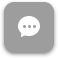Call Now 855-300-1469

# BUS 5446 Investment Management

#### Course Description

Investigates the concepts, theories and techniques underlying the development of investment policies and strategies.

### Week 1

##### Lecture: Stock-Trak Portfolio

Outcomes

• Discuss the importance of financial assets in the economy
• Explain the role of financial markets, investment bankers and financial intermediaries
• Differentiate between asset classes
• Demonstrate how to calculate rate of return on a market index

### Week 2

##### Lecture: Trading on Margin and Selling Short

Outcomes

• Define how financial markets operate
• Differentiate between the types of orders
• Demonstrate knowledge of margin accounts and short sales
• Identify the different types of mutual funds and other investment companies

### Week 3

##### Lecture: Risk-Return Tradeoff and Capital Allocation

Outcomes

• Discuss the historical risk-return relationship in financial markets
• Differentiate between arithmetic and geometric mean
• Explain skewness, kurtosis and normal distribution
• Discuss risk aversion
• Demonstrate how to allocate portfolio assets and how to calculate portfolio expected return and standard deviation

### Week 4

##### Lecture: Optimal Risky Portfolios

Outcomes

• Understand diversifying portfolio risk
• Learn Markowitz’s portfolio theory
• Discuss the uses of a single-index model to construct a portfolio
• Understand how portfolio optimization works

### Week 5

##### Lecture: Technical Analysis

Outcomes

• Define the limitations of CAPM
• Explain Arbitrage Pricing Theory
• Explore multi-factor models and reflect on what those factors should be
• Discuss introductory concepts of behavioral finance and technical analysis
• Calculate technical indicators

### Week 6

##### Lecture: Term Structure of Interest Rates

Outcomes

• Define the term structure of interest rates
• Calculate yield to maturity (YTM) and forward rates
• Discuss the uses of options to manage portfolio risk
• Calculate the rate of return on investing in options
• Explain the put-call parity relationship

### Week 7

##### Lecture: Options and Futures Valuation

Outcomes

• Use the Black-Scholes formula to value options
• Apply the put-call parity relationship
• Explain how futures trade in the market
• Identify if an arbitrage opportunity exists for an asset
• Calculate the profits of a futures transaction

### Week 8

##### Lecture: International Diversification

Outcomes

• Calculate performance measures
• Calculate time-weighted and dollar-weighted average returns
• Explain the risk-return tradeoffs of international investing
• Discuss the impact of international diversification

The course description, objectives and learning outcomes are subject to change without notice based on enhancements made to the course. January 2012